# 图像处理 高斯模糊

## 图像处理------高斯模糊

2015-07-24 09:32:44 mao0514 阅读数 1884
• ###### Python+OpenCV3.3图像处理视频教程

Python+OpenCV3.3图像处理视频培训课程：该教程基于Python3.6+OpenCV新版本3.3.0详细讲述Python OpenCV图像处理部分内容，包括opencv人脸识别、人脸检测、数字验证码识别等内容。是Python开发者学习图像知识与应用...

30课时 663分钟 6356人学习 贾志刚
免费试看1. private float[] get1DKernalData(int n, float sigma) {
2.     float sigma22 = 2*sigma*sigma;
3.     float Pi2 = 2*(float)Math.PI;
4.     float sqrtSigmaPi2 = (float)Math.sqrt(Pi2) * sigma ;
5.     int size = 2*n + 1;
6.     int index = 0;
7.     float[] kernalData = new float[size];
8.     for(int i=-n; i<=n; i++) {
9.         float distance = i*i;
10.         kernalData[index] = (float)Math.exp((-distance)/sigma22)/sqrtSigmaPi2;
11.         System.out.println("\t" + kernalData[index]);
12.         index++;
13.     }
14.     return kernalData;
15. }

0.24197073, 0.3989423,0.241970731. public float[][] get2DKernalData(int n, float sigma) {
2.     int size = 2*n +1;
3.     float sigma22 = 2*sigma*sigma;
4.     float sigma22PI = (float)Math.PI * sigma22;
5.     float[][] kernalData = new float[size][size];
6.     int row = 0;
7.     for(int i=-n; i<=n; i++) {
8.         int column = 0;
9.         for(int j=-n; j<=n; j++) {
10.             float xDistance = i*i;
11.             float yDistance = j*j;
12.             kernalData[row][column] = (float)Math.exp(-(xDistance + yDistance)/sigma22)/sigma22PI;
13.             column++;
14.         }
15.         row++;
16.     }
17.
18.     for(int i=0; i<size; i++) {
19.         for(int j=0; j<size; j++) {
20.             System.out.print("\t" + kernalData[i][j]);
21.         }
22.         System.out.println();
23.         System.out.println("\t ---------------------------");
24.     }
25.     return kernalData;
26. }

0.058549833   0.09653235     0.058549833

0.09653235     0.15915494     0.09653235

0.058549833   0.09653235     0.0585498331. 生成高斯操作数即Kernel Data

2. 从图像中读取像素，利用第一步的操作数，完成卷积。

3. 发现图像处理前后的最大像素值peak得出rate

4. 完成归一化操作，返回处理后像素数组

1.     // normalization
2.     float rate = inMax/outMax;
3.     System.out.println("Rate = " + rate);
4.     for(int row=0; row<height; row++) {
5.         for(int col=0; col<width; col++) {
6.             index = row * width + col;
7.             int rgb1 = tempoutPixels[index];
8. int red = (rgb1 >> 16) & 0xff;
9. int green = (rgb1 >> 8) & 0xff;
10. int blue = rgb1 & 0xff;
11. red = (int)(rate * red);
12. green = (int)(rate * green);
13. blue = (int)(rate * blue);
14. outPixels[index] = (rgb1 & 0xff000000) | (red << 16) | (green << 8) | blue;
15.         }
16.     }- 左边为原图                                                                                                                                                            - 右边为高斯模糊之后效果，发现皱纹和手部滑了1. int index = 0;
2. for ( int y = 0; y < height; y++ ) {
3.     for ( int x = 0; x < width; x++ ) {
4.         int rgb1 = outPixels[index];
5.         int r1 = (rgb1 >> 16) & 0xff;
6.         int g1 = (rgb1 >> 8) & 0xff;
7.         int b1 = rgb1 & 0xff;
8.
9.         int rgb2 = inPixels[index];
10.         int r2 = (rgb2 >> 16) & 0xff;
11.         int g2 = (rgb2 >> 8) & 0xff;
12.         int b2 = rgb2 & 0xff;
13.
14.         r1 = PixelUtils.clamp( (int)(r1 + a * r2) );
15.         g1 = PixelUtils.clamp( (int)(g1 + a * g2) );
16.         b1 = PixelUtils.clamp( (int)(b1 + a * b2) );
17.
18.         inPixels[index] = (rgb1 & 0xff000000) | (r1 << 16) | (g1 << 8) | b1;
19.         index++;
20.     }
21. }

## opencv图像处理05-高斯模糊和均值模糊

2018-07-29 11:59:10 qinchao315 阅读数 9736
• ###### Python+OpenCV3.3图像处理视频教程

Python+OpenCV3.3图像处理视频培训课程：该教程基于Python3.6+OpenCV新版本3.3.0详细讲述Python OpenCV图像处理部分内容，包括opencv人脸识别、人脸检测、数字验证码识别等内容。是Python开发者学习图像知识与应用...

30课时 663分钟 6356人学习 贾志刚
免费试看

### 模糊原理：

1. 模糊原理和上几节说的图像掩模矩阵有很多相似的地方，都是拿一个矩阵（3X3, 5X5）等，和原图从左向右从上到下分别进行卷积，将卷积值最后赋值个当前卷积的中心像素。

2. 那么其最关键的参数，也就在于矩阵的大小和矩阵的值，我们通常称矩阵为卷积核。

3. 模糊操作的重要原因之一也是为了给图像预处理时降低噪声。

### 卷积示意图：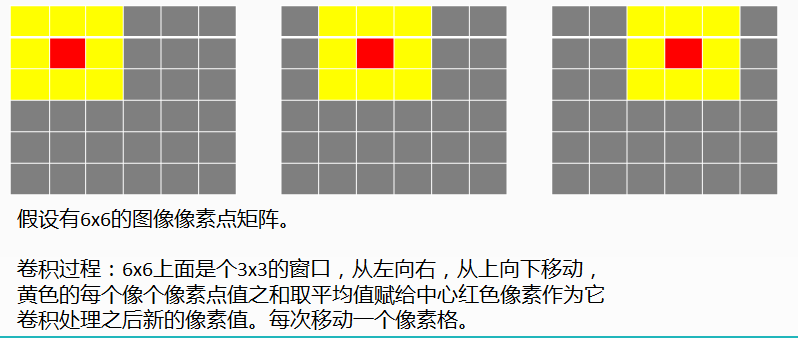### 均值模糊：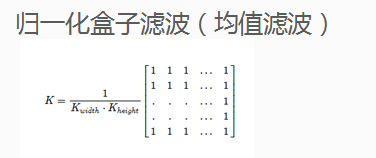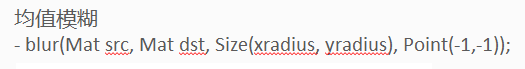### 高斯模糊：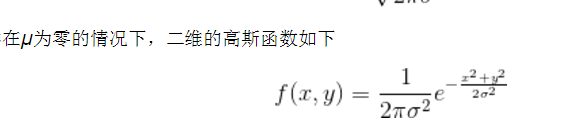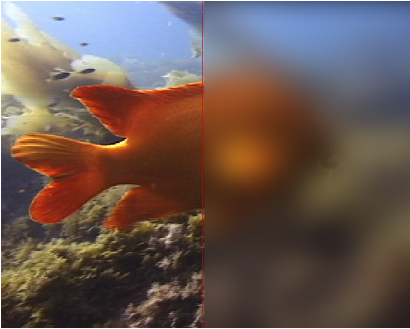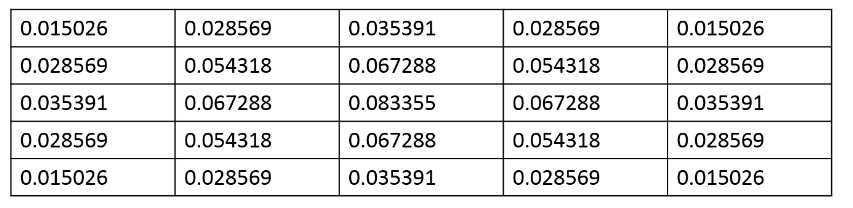### 代码演示：

``````#include <opencv2/opencv.hpp>
#include <iostream>
using namespace cv;

int main(int argc, char** argv) {
Mat src, dst;
if (!src.data) {
return -1;
}
char input_title[] = "input image";
char output_title[] = "blur image";
namedWindow(input_title, CV_WINDOW_AUTOSIZE);
namedWindow(output_title, CV_WINDOW_AUTOSIZE);
imshow(input_title, src);

//均值模糊API
blur(src, dst, Size(11, 11), Point(-1, -1));
imshow(output_title, dst);

Mat gblur;
//高斯模糊API
GaussianBlur(src, gblur, Size(11, 11), 4, 4);
imshow("gaussian blur", gblur);

waitKey(0);
return 0;
}``````

## 图像处理（7）--高斯模糊原理

2019-07-10 17:04:33 qq_33208851 阅读数 3140
• ###### Python+OpenCV3.3图像处理视频教程

Python+OpenCV3.3图像处理视频培训课程：该教程基于Python3.6+OpenCV新版本3.3.0详细讲述Python OpenCV图像处理部分内容，包括opencv人脸识别、人脸检测、数字验证码识别等内容。是Python开发者学习图像知识与应用...

30课时 663分钟 6356人学习 贾志刚
免费试看

### 文章目录结构

图像处理系列笔记： https://blog.csdn.net/qq_33208851/article/details/95335809

## 2. 高斯模糊原理

### 2.1 矩阵范围的像素平均值

“模糊”，就是将图像中每个像素值进行重置的过程，这个过程采用将每一个像素都设置成周边像素的平均值。上图中，2是中间点，周边点都是1。假设周边的点对中间点的影响都是相同的，即构造的卷积算子如下：然后计算求和：将图中‘2’置为：“中间点"取"周围点"的平均值，变成10/9。在数值上，这是一种"平滑化”。在图形上，就相当于产生"模糊"效果，"中间点"失去细节。
显然，计算平均值时，取值范围越大，"模糊效果"越强烈。如下三幅图，分别表示原图，3X3和5X5的模糊效果图。接下来的问题就是，既然每个点都要取周边像素的平均值，那么应该如何分配权重呢？
如果使用简单平均，显然不是很合理，因为图像都是连续的，越靠近的点关系越密切，越远离的点关系越疏远。因此，加权平均更合理，距离越近的点权重越大，距离越远的点权重越小。

### 2.2 正态分布的权重

正态分布显然是一种可取的权重分配模式。
在图形上，正态分布是一种钟形曲线，越接近中心，取值越大，越远离中心，取值越小。
计算平均值的时候，我们只需要将"中心点"作为原点，其他点按照其在正态曲线上的位置，分配权重，就可以得到一个加权平均值。
高斯函数正态分布的密度函数叫做"高斯函数"（Gaussian function）。它的一维形式是：其中，μ是x的均值，σ是x的方差。因为计算平均值的时候，中心点就是原点，所以μ等于0。上面的正态分布是一维的，图像都是二维的，所以我们需要二维的正态分布。有了这个函数 ，就可以计算每个点的权重了。
权重矩阵：
假定中心点的坐标是（0,0），那么距离它最近的8个点的坐标如下：更远的点以此类推。
为了计算权重矩阵，需要设定σ的值。假定σ=1.5（σ值越大图像越平滑/模糊），则模糊半径为1的权重矩阵如下：这9个点的权重总和等于0.4787147，如果只计算这9个点的加权平均，还必须让它们的权重之和等于1，因此上面9个值还要分别除以0.4787147，得到最终的权重矩阵## 3. 计算高斯模糊

有了权重矩阵，就可以计算高斯模糊的值了。假设现有9个像素点，灰度值（0-255）如下：每个点乘以自己的权重值：将这9个值加起来，就是中心点的高斯模糊的值。
对所有点重复这个过程，就得到了高斯模糊后的图像。如果原图是彩色图片，可以对RGB三个通道分别做高斯模糊。

图像处理 高斯模糊 相关内容

## 图像处理之高斯模糊

2012-02-05 14:51:15 jia20003 阅读数 28706
• ###### Python+OpenCV3.3图像处理视频教程

Python+OpenCV3.3图像处理视频培训课程：该教程基于Python3.6+OpenCV新版本3.3.0详细讲述Python OpenCV图像处理部分内容，包括opencv人脸识别、人脸检测、数字验证码识别等内容。是Python开发者学习图像知识与应用...

30课时 663分钟 6356人学习 贾志刚
免费试看

高斯模糊是一种两维的卷积模糊操作，在图像完成高斯模糊相对于均值模糊来说，

计算量会增加，但是高斯模糊可以实现一些特殊效果，特别是在图像噪声(非椒盐

噪声)消去方面，更是有着非常好的效果。一维高斯公式如下：其中x是制定[-n,n]范围的整数，sigma代表标准方差。通常取值为1.

一维高斯函数Java代码如下：

```	private float[] get1DKernalData(int n, float sigma) {
float sigma22 = 2*sigma*sigma;
float Pi2 = 2*(float)Math.PI;
float sqrtSigmaPi2 = (float)Math.sqrt(Pi2) * sigma ;
int size = 2*n + 1;
int index = 0;
float[] kernalData = new float[size];
for(int i=-n; i<=n; i++) {
float distance = i*i;
kernalData[index] = (float)Math.exp((-distance)/sigma22)/sqrtSigmaPi2;
System.out.println("\t" + kernalData[index]);
index++;
}
return kernalData;
}```

假设输入 n= 1, sigma = 1时，输出的Kernel数据为：

0.24197073, 0.3989423, 0.24197073

两维的高斯分布函数为：对应的Java实现代码为：

```	public float[][] get2DKernalData(int n, float sigma) {
int size = 2*n +1;
float sigma22 = 2*sigma*sigma;
float sigma22PI = (float)Math.PI * sigma22;
float[][] kernalData = new float[size][size];
int row = 0;
for(int i=-n; i<=n; i++) {
int column = 0;
for(int j=-n; j<=n; j++) {
float xDistance = i*i;
float yDistance = j*j;
kernalData[row][column] = (float)Math.exp(-(xDistance + yDistance)/sigma22)/sigma22PI;
column++;
}
row++;
}

for(int i=0; i<size; i++) {
for(int j=0; j<size; j++) {
System.out.print("\t" + kernalData[i][j]);
}
System.out.println();
System.out.println("\t ---------------------------");
}
return kernalData;
}```

当n=1, sigma=1时对应输出的Kernel数据为：

0.058549833   0.09653235     0.058549833

0.09653235     0.15915494     0.09653235

0.058549833   0.09653235     0.058549833

一个2D高斯分布的图可以表示如下：高斯过滤在图像处理是一种低通滤波，会除去图像的细节而保持整体不变化，在图像美化和特效

方面，高斯过滤有这很多应用。高斯模糊不同于均值模糊！

本文实现完整的高斯模糊算法包括下面几个步骤：

1. 生成高斯操作数即Kernel Data

2. 从图像中读取像素，利用第一步的操作数，完成卷积。

3. 发现图像处理前后的最大像素值peak得出rate

4. 完成归一化操作，返回处理后像素数组

关键程序解析：

利用操作数完成卷积的代码参看以前的Blog文章《图像处理之理解卷积

完成归一化操作的算法非常简单, 主要是利用第三步计算出来的rate

```        // normalization
float rate = inMax/outMax;
System.out.println("Rate = " + rate);
for(int row=0; row<height; row++) {
for(int col=0; col<width; col++) {
index = row * width + col;
int rgb1 = tempoutPixels[index];
int red = (rgb1 >> 16) & 0xff;
int green = (rgb1 >> 8) & 0xff;
int blue = rgb1 & 0xff;
red = (int)(rate * red);
green = (int)(rate * green);
blue = (int)(rate * blue);
outPixels[index] = (rgb1 & 0xff000000) | (red << 16) | (green << 8) | blue;
}
}```

高斯模糊效果如下：- 左边为原图                                                                                                                                                            - 右边为高斯模糊之后效果，发现皱纹和手部滑了

等等现在还不最cool的效果，高斯模糊之后如果与原图像叠加会出现一种Glow的

效果，好像灯光打在图像上一样，Glow处理之后的运行效果如下：

原图：实现Glow Filter之后的图像：实现Glow算法只是高斯模糊输出像素值叠加原来的像素值。

```		int index = 0;
for ( int y = 0; y < height; y++ ) {
for ( int x = 0; x < width; x++ ) {
int rgb1 = outPixels[index];
int r1 = (rgb1 >> 16) & 0xff;
int g1 = (rgb1 >> 8) & 0xff;
int b1 = rgb1 & 0xff;

int rgb2 = inPixels[index];
int r2 = (rgb2 >> 16) & 0xff;
int g2 = (rgb2 >> 8) & 0xff;
int b2 = rgb2 & 0xff;

r1 = PixelUtils.clamp( (int)(r1 + a * r2) );
g1 = PixelUtils.clamp( (int)(g1 + a * g2) );
b1 = PixelUtils.clamp( (int)(b1 + a * b2) );

inPixels[index] = (rgb1 & 0xff000000) | (r1 << 16) | (g1 << 8) | b1;
index++;
}
}```

转载时请注明出处！！，谢谢！

图像处理 高斯模糊 相关内容

## 图像处理：高斯模糊

2018-08-20 14:16:47 qinghuaci666 阅读数 3101
• ###### Python+OpenCV3.3图像处理视频教程

Python+OpenCV3.3图像处理视频培训课程：该教程基于Python3.6+OpenCV新版本3.3.0详细讲述Python OpenCV图像处理部分内容，包括opencv人脸识别、人脸检测、数字验证码识别等内容。是Python开发者学习图像知识与应用...

30课时 663分钟 6356人学习 贾志刚
免费试看

通常，图像处理软件会提供"模糊"（blur）滤镜，使图片产生模糊的效果。"模糊"的算法有很多种，其中有一种叫做"高斯模糊"（Gaussian Blur）。它将正态分布（又名"高斯分布"）用于图像处理。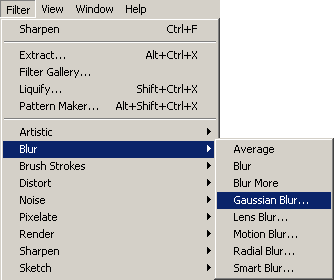本文介绍"高斯模糊"的算法，你会看到这是一个非常简单易懂的算法。本质上，它是一种数据平滑技术（data smoothing），适用于多个场合，图像处理恰好提供了一个直观的应用实例。

一、高斯模糊的原理

所谓"模糊"，可以理解成每一个像素都取周边像素的平均值。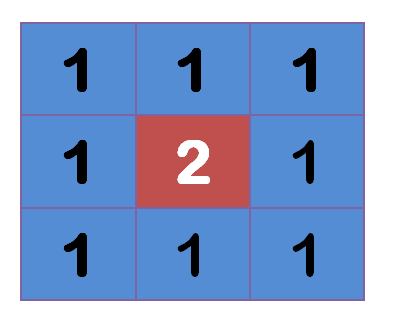上图中，2是中间点，周边点都是1。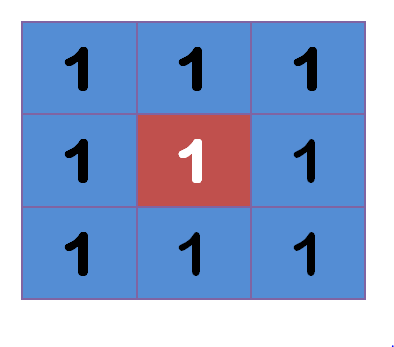"中间点"取"周围点"的平均值，就会变成1。在数值上，这是一种"平滑化"。在图形上，就相当于产生"模糊"效果，"中间点"失去细节。显然，计算平均值时，取值范围越大，"模糊效果"越强烈。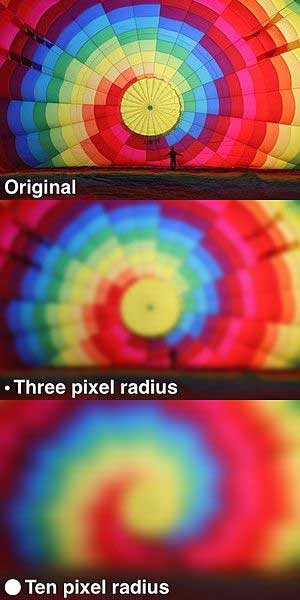上面分别是原图、模糊半径3像素、模糊半径10像素的效果。模糊半径越大，图像就越模糊。从数值角度看，就是数值越平滑。

接下来的问题就是，既然每个点都要取周边像素的平均值，那么应该如何分配权重呢？

如果使用简单平均，显然不是很合理，因为图像都是连续的，越靠近的点关系越密切，越远离的点关系越疏远。因此，加权平均更合理，距离越近的点权重越大，距离越远的点权重越小。

二、正态分布的权重

正态分布显然是一种可取的权重分配模式。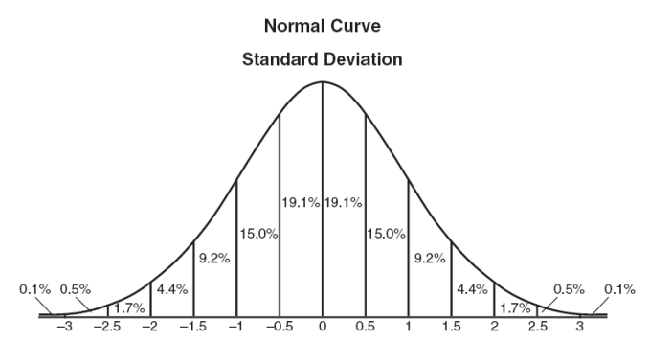在图形上，正态分布是一种钟形曲线，越接近中心，取值越大，越远离中心，取值越小。

计算平均值的时候，我们只需要将"中心点"作为原点，其他点按照其在正态曲线上的位置，分配权重，就可以得到一个加权平均值。

三、高斯函数

上面的正态分布是一维的，图像都是二维的，所以我们需要二维的正态分布。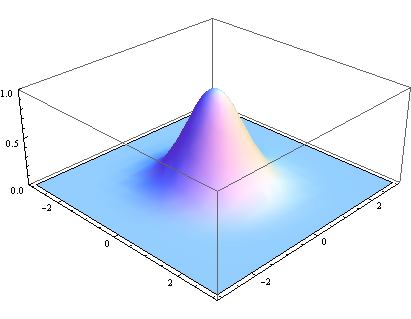正态分布的密度函数叫做"高斯函数"（Gaussian function）。它的一维形式是：其中，μ是x的均值，σ是x的方差。因为计算平均值的时候，中心点就是原点，所以μ等于0。根据一维高斯函数，可以推导得到二维高斯函数：有了这个函数 ，就可以计算每个点的权重了。

四、权重矩阵

假定中心点的坐标是（0,0），那么距离它最近的8个点的坐标如下：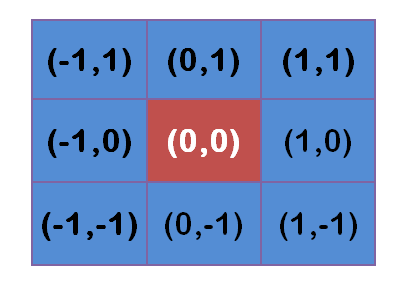更远的点以此类推。

为了计算权重矩阵，需要设定σ的值。假定σ=1.5，则模糊半径为1的权重矩阵如下：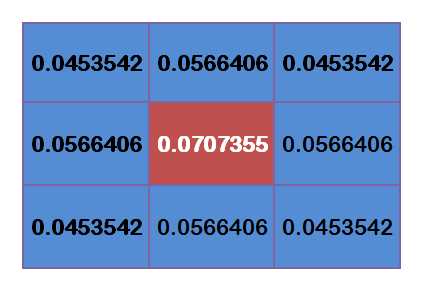这9个点的权重总和等于0.4787147，如果只计算这9个点的加权平均，还必须让它们的权重之和等于1，因此上面9个值还要分别除以0.4787147，得到最终的权重矩阵。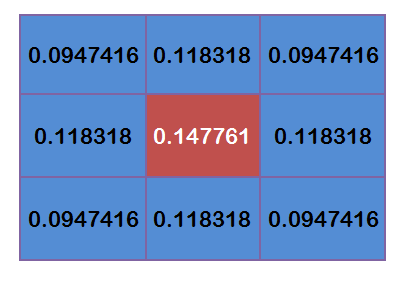五、计算高斯模糊

有了权重矩阵，就可以计算高斯模糊的值了。

假设现有9个像素点，灰度值（0-255）如下：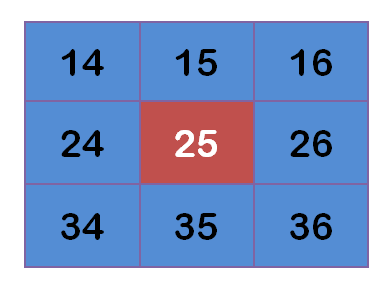每个点乘以自己的权重值：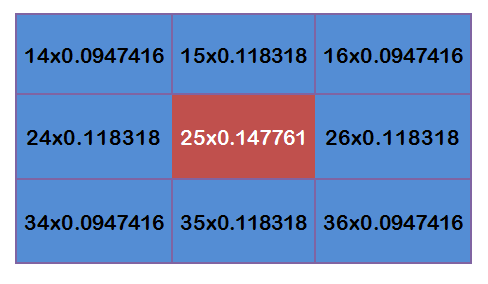得到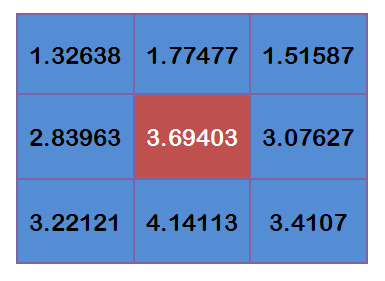将这9个值加起来，就是中心点的高斯模糊的值。

对所有点重复这个过程，就得到了高斯模糊后的图像。如果原图是彩色图片，可以对RGB三个通道分别做高斯模糊。

六、边界点的处理

如果一个点处于边界，周边没有足够的点，怎么办？

一个变通方法，就是把已有的点拷贝到另一面的对应位置，模拟出完整的矩阵。

参考文献

图像处理 高斯模糊 相关内容

阅读数 9127

阅读数 726

阅读数 778

阅读数 4455